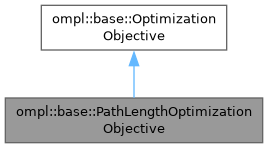ompl::base::PathLengthOptimizationObjective Class Reference

An optimization objective which corresponds to optimizing path length. More...

#include <ompl/base/objectives/PathLengthOptimizationObjective.h>

Inheritance diagram for ompl::base::PathLengthOptimizationObjective:[legend]

## Public Member Functions

PathLengthOptimizationObjective (const SpaceInformationPtr &si)

Cost stateCost (const State *s) const override
Returns identity cost.

Cost motionCost (const State *s1, const State *s2) const override
Motion cost for this objective is defined as the configuration space distance between s1 and s2, using the method SpaceInformation::distance().

Cost motionCostHeuristic (const State *s1, const State *s2) const override
the motion cost heuristic for this objective is simply the configuration space distance between s1 and s2, since this is the optimal cost between any two states assuming no obstacles.

InformedSamplerPtr allocInformedStateSampler (const ProblemDefinitionPtr &probDefn, unsigned int maxNumberCalls) const override
Allocate a state sampler for the path-length objective (i.e., direct ellipsoidal sampling).Public Member Functions inherited from ompl::base::OptimizationObjective
OptimizationObjective (const OptimizationObjective &)=delete

OptimizationObjectiveoperator= (const OptimizationObjective &)=delete

OptimizationObjective (SpaceInformationPtr si)
Constructor. The objective must always know the space information it is part of. The cost threshold for objective satisfaction defaults to 0.0.

const std::string & getDescription () const
Get the description of this optimization objective.

virtual bool isSatisfied (Cost c) const
Check if the the given cost c satisfies the specified cost objective, defined as better than the specified threshold.

Cost getCostThreshold () const
Returns the cost threshold currently being checked for objective satisfaction.

void setCostThreshold (Cost c)
Set the cost threshold for objective satisfaction. When a path is found with a cost better than the cost threshold, the objective is considered satisfied.

virtual bool isCostBetterThan (Cost c1, Cost c2) const
Check whether the the cost c1 is considered better than the cost c2. By default, this returns true if if c1 is less than c2.

virtual bool isCostEquivalentTo (Cost c1, Cost c2) const
Compare whether cost c1 and cost c2 are equivalent. By default defined as !isCostBetterThan(c1, c2) && !isCostBetterThan(c2, c1), as if c1 is not better than c2, and c2 is not better than c1, then they are equal.

virtual bool isFinite (Cost cost) const
Returns whether the cost is finite or not.

virtual Cost betterCost (Cost c1, Cost c2) const
Return the minimum cost given c1 and c2. Uses isCostBetterThan.

virtual Cost controlCost (const control::Control *c, unsigned int steps) const
Get the cost that corresponds to the motion created by a control c applied for duration steps. The default implementation uses the identityCost.

virtual Cost combineCosts (Cost c1, Cost c2) const
Get the cost that corresponds to combining the costs c1 and c2. Default implementation defines this combination as an addition.

virtual Cost identityCost () const
Get the identity cost value. The identity cost value is the cost c_i such that, for all costs c, combineCosts(c, c_i) = combineCosts(c_i, c) = c. In other words, combining a cost with the identity cost does not change the original cost. By default, a cost with the value 0.0 is returned. It's very important to override this with the proper identity value for your optimization objectives, or else optimal planners may not work.

virtual Cost infiniteCost () const
Get a cost which is greater than all other costs in this OptimizationObjective; required for use in Dijkstra/Astar. Defaults to returning the double value inf.

virtual Cost initialCost (const State *s) const
Returns a cost value corresponding to starting at a state s. No optimal planners currently support this method. Defaults to returning the objective's identity cost.

virtual Cost terminalCost (const State *s) const
Returns a cost value corresponding to a path ending at a state s. No optimal planners currently support this method. Defaults to returning the objective's identity cost.

virtual bool isSymmetric () const
Check if this objective has a symmetric cost metric, i.e. motionCost(s1, s2) = motionCost(s2, s1). Default implementation returns whether the underlying state space has symmetric interpolation.

virtual Cost averageStateCost (unsigned int numStates) const
Compute the average state cost of this objective by taking a sample of numStates states.

void setCostToGoHeuristic (const CostToGoHeuristic &costToGo)
Set the cost-to-go heuristic function for this objective. The cost-to-go heuristic is a function which returns an admissible estimate of the optimal path cost from a given state to a goal, where "admissible" means that the estimated cost is always less than the true optimal cost.

bool hasCostToGoHeuristic () const
Check if this objective has a cost-to-go heuristic function.

Cost costToGo (const State *state, const Goal *goal) const
Uses a cost-to-go heuristic to calculate an admissible estimate of the optimal cost from a given state to a given goal. If no cost-to-go heuristic has been specified with setCostToGoHeuristic(), this function just returns the identity cost, which is sure to be an admissible heuristic if there are no negative costs.

const SpaceInformationPtrgetSpaceInformation () const
Returns this objective's SpaceInformation. Needed for operators in MultiOptimizationObjective.

virtual void print (std::ostream &out) constProtected Attributes inherited from ompl::base::OptimizationObjective
SpaceInformationPtr si_
The space information for this objective.

std::string description_
The description of this optimization objective.

Cost threshold_
The cost threshold used for checking whether this objective has been satisfied during planning.

CostToGoHeuristic costToGoFn_
The function used for returning admissible estimates on the optimal cost of the path between a given state and goal.

## Detailed Description

An optimization objective which corresponds to optimizing path length.

Definition at line 111 of file PathLengthOptimizationObjective.h.

The documentation for this class was generated from the following files: